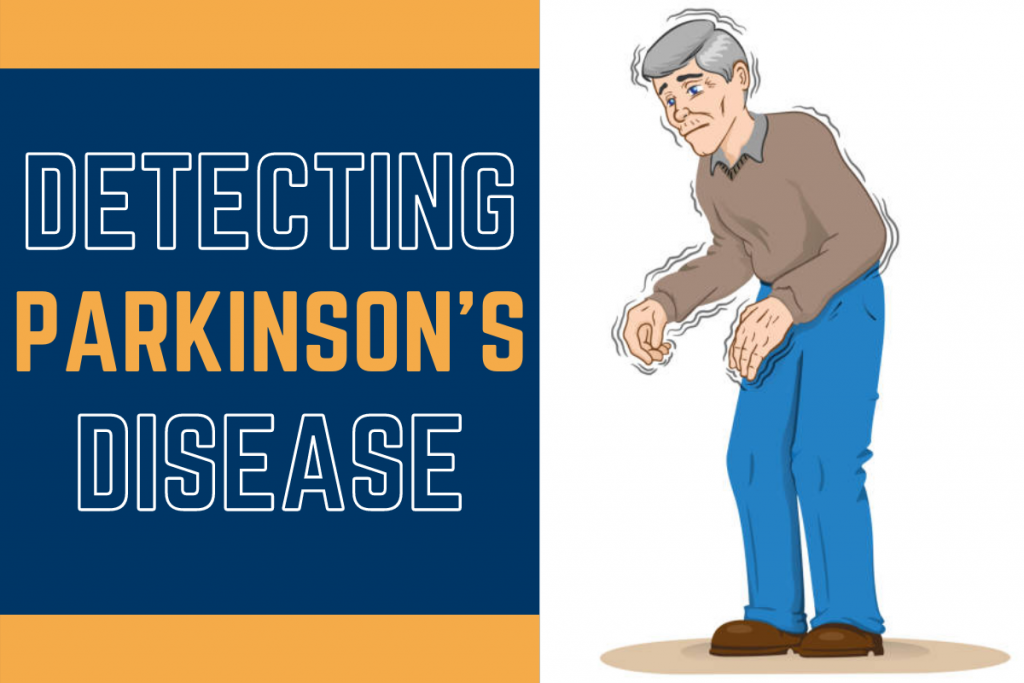# Detecting Parkinson’s Disease using PythonHello, there fellow learner! Today we’re building a basic ML model to detect Parkinson’s Disease based on some pre-acquired information using Python.

So let’s begin by first understanding Parkinson’s Disease and the dataset we will be using for our model, which can be found here. We will be using the `parkinson.data` file for our project.

Parkinson’s disease is a disorder in the central nervous system which affects the movement of the body. Till now there is no practical cure for the disease.

## Importing the Required Libraries

The first step of any project is to import all the necessary modules into our project. We would require some basic modules like numpy, pandas, and matplotlib to prepare, load, and plot data respectively.

Then we also require some sklearn models and functions for training and estimating accuracy. Last but not least, we would be using the `xgboost` library.

XGBoost library is a decision tree-based Gradient Boosting model designed to increase the speed and accuracy of the system.

```import numpy as np
import pandas as pd
import os, sys
from sklearn.preprocessing import MinMaxScaler
from xgboost import XGBClassifier
from sklearn.model_selection import train_test_split
from sklearn.metrics import accuracy_score
```

The next step is to load the data that we downloaded earlier into same folder as the code file. For the same we make use of the pandas module and the code for the same is shown below.

```dataframe=pd.read_csv('parkinsons.csv')
print("The shape of data is: ",dataframe.shape,"\n")
print("FIRST FIVE ROWS OF DATA ARE AS FOLLOWS: \n")
```

The output of the program displays the first five rows of the dataset which consists of a total of 24 columns and 195 data points. The next step is to separate the labels and the data from each other.

The code for the same is mentioned below. Here the label column is the status column.

```data=dataframe.loc[:,dataframe.columns!='status'].values[:,1:]
label=dataframe.loc[:,'status'].values
```

## Normalizing the data

The next step is scaling all the data points between -1 and +1. We would be using MinMaxScaler to transform features and scale them to a given range as a parameter. The `fit_transform` function helps to fit the data and then transform/normalize it.

Scaling of labels is not required as they already have only two values i.e. 0 and 1. The code for the same is shown below.

```Normalizing_object = MinMaxScaler((-1,1))
x_data = Normalizing_object.fit_transform(data)
y_data=label
```

## Train-Test Split of data

The next step is to split the data into training and testing data according to the 80-20 rule where 80% of data goes to training and the rest 20% to testing.

We will be using the `train_test_split` function of the sklearn module to achieve the same. The code is mentioned below.

```x_train,x_test,y_train,y_test=train_test_split(x_data,y_data,test_size=0.2)
```

## Initializing the XGBClassifier and training of the modek

Our data is now ready to get trained and fit into the XBGClassifier. To do the same, we are going to create a classifier object and then fit the training data into the classifier.

The code for the same is shown below.

```model=XGBClassifier()
model.fit(x_train,y_train)
```

The output displays the whole training information of the Classifier and now we are ready to make predictions for the testing data and then get accuracy.

## Get predictions and accuracy

The next and the final step is to get predictions for the testing dataset and estimating the accuracy of our model. The code to do the same is shown below.

```predictions=model_obj.predict(x_test)
print(accuracy_score(y_test,predictions)*100)
```

After running the code we come to know that the model is over `97.43%` accurate which is pretty good right?! So there we go! We build our own Parkinson’s Disease Classifier.

## Conclusion

In this tutorial, we learned how to detect the presence of Parkinson’s Disease in individuals according to various factors.

For the project, we made use of the XGBClassifier for fast and accurate detection. The model gave us an accuracy of over `97.43%`, which is great!